Search
•Aditya Anand

# Breaking Down : SHA-512 Algorithm

#### Looking under the hood and understanding how it works?I have been writing the “Breaking Down” series for past few weeks now, where I explain hashing algorithms in extreme detail so that anyone reading it can understand every bit of operation taking place to create a hash output.

Breaking Down : The series

1. Breaking Down : MD5 Algorithm

2. Breaking Down: SHA-1 Algorithm

This is the third part of the series where I try to explain SHA-512 algorithm and why it is still unbreakable while SHA-1 and MD5 has been cracked already.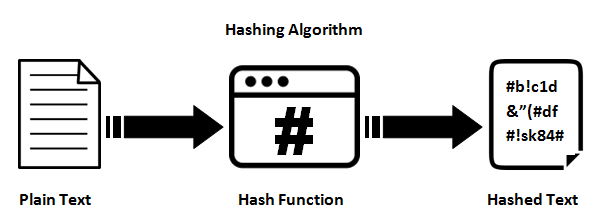### Let’s Begin!

So, I will break down the entire working of the algorithm in a step by step process. The overall functioning of SHA-512 is very similar to SHA-256 ( most probably my next article ), so if you already know about it, understanding the SHA-512 algorithm will be really simple.

#### 1. Append : Padding bits

The first step is to carry out the padding function in which we append a certain number of bits to the plaintext message to increase its length, which should be exactly 128 bits less than an exact multiple of 1024.

When we are appending these bits at the end of the message we start with a ‘1’ and then keep on adding ‘0’ till the moment we reach the last bit that needs to be appended under padding and leave the 128 bits after that.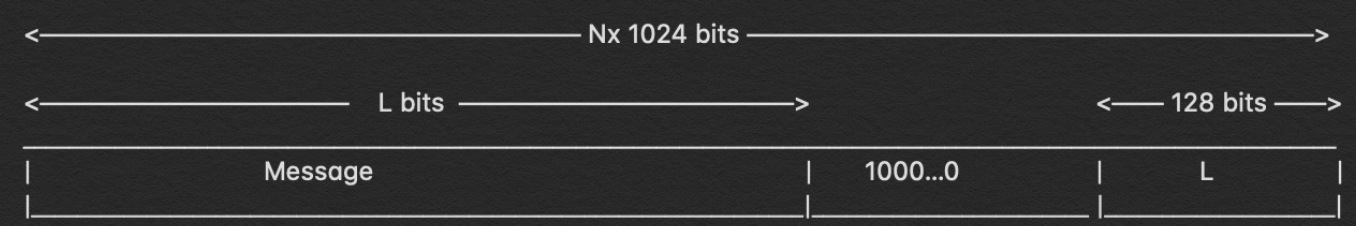#### 2. Append : Length bits

Now, we add the remaining 128 bits left to this entire block to make it an exact multiple of 1024, so that the whole thing can be broken down in ’n’ number of 1024 blocks of message that we will apply our operation on. The way to calculate the rest of the 128 bits is by calculating the modulo with 2⁶⁴.

Once done, we append it to the padded bit and the original message to make the entire length of the block to be “n x 1024” in length.

#### 3. Initialize the buffers

Now, that we have “n x 1024” length bit message that we need to hash, let us focus on the parts of the hashing function itself. To carry on the hash and the computations required, we need to have some default values initialized.

```a = 0x6a09e667f3bcc908
b = 0xbb67ae8584caa73b
c = 0x3c6ef372fe94f82b
d = 0xa54ff53a5f1d36f1
f = 0x9b05688c2b3e6c1f
g = 0x1f83d9abfb41bd6b
h = 0x5be0cd19137e2179```

These are the values of the buffer that we will need, there are other default values that we need to initialize as well. These are the value for the ‘k’ variable which we will be using.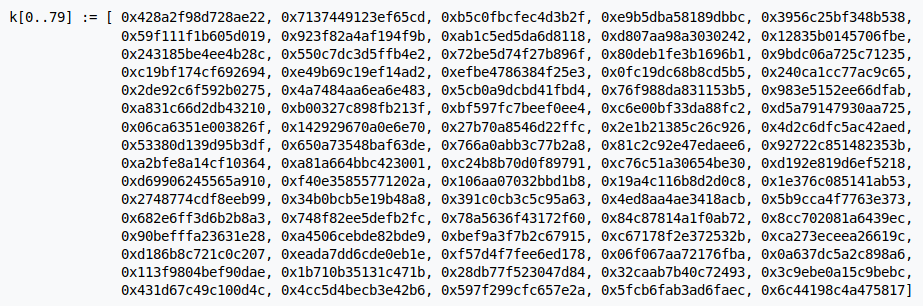The reason for initiating these values will be clear to you in the very next step that we will explore.

#### 4. Compression function

Now, we need to have a wider look at the hash function so that we can understand what is happening. First of all, we take 1024 bits of messages and divide the complete message in ‘n’ bits.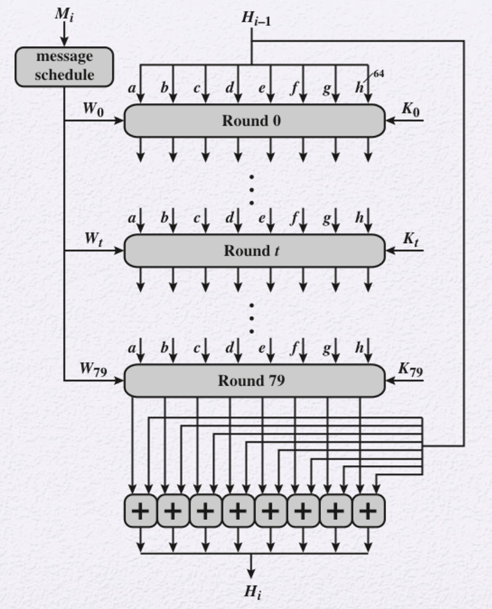Having a look at the above image you get the idea that we will be working on 80 rounds and the input, W will be derived from the 1024 bits, which is further divided into 16 parts. The value of W from 0 to 15 is the message in plaintext but the values of W from 16 to 79 is calculated from the previous 16 blocks and we have the formula mentioned below.

```W(t) = σ¹(Wᵗ⁻²) + Wᵗ⁻⁷ + σ⁰(Wᵗ⁻¹⁵) + Wᵗ⁻¹⁶
where,
σ⁰(x)    = ROTR¹(x) ϕ ROTR⁸(x) ϕ SHR⁷(x)
σ¹(x)    = ROTR¹⁹(x) ϕ ROTR⁶¹(x) ϕ SHR⁶(x)
ROTRⁿ(x) = Circular right rotation of 'x' by 'n' bits
SHRⁿ(x)  = Circular right shift of 'x' by 'n' bits

Now that we know the methodology to obtain the values of W for 0 to 79 and already have the values for K as well for all the rounds from 0 to 79 we can proceed ahead and see where and how we do put in these values for the computation of the hash.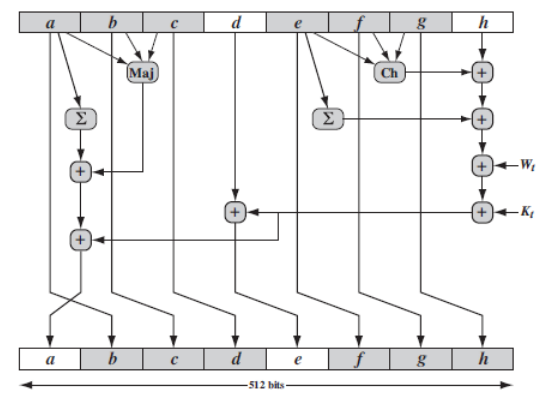In the image above we can see exactly what happens in each round and now that we have the values and formulas for each of the functions carried out we can perform the entire hashing process.

```Ch(E, F, G) = (E AND F) XOR ((NOT E) AND G)
Ma(A, B, C) = (A AND B) XOR (A AND C) XOR (B AND C)
∑(A)    = (A >>> 2) XOR (A >>> 13) XOR (A >>> 22)
∑(E)    = (E >>> 6) XOR (E >>> 11) XOR (E >>> 25)

These are the functions that are performed in each of the 80 rounds that are performed over and over for ‘n’ number of times.

#### 5. Output

The output from every round act as an input for the next round and this process keeps on continuing till the last bit of the message go through one of the rounds and the output from the last block of the last message is the hash code. The length of the output is 512 bits.

### Conclusion

The SHA-512 hashing algorithm is currently one of the best and secured hashing algorithms after hashes like MD5 and SHA-1 has been broken down. Due to their complicated nature, it is not well accepted and SHA-256 is a general standard, but the industry is slowing moving towards this hashing algorithm.

Let’s have a look at the entire working of the SHA-512 hashing algorithm and see in a concise manner how it works.

The message that needs to be hashed, we first append a few bits to it, making it exactly 128 bits less than the multiple of 1024. The remaining 128 bits are added after we calculate the modulo of the original message with 2⁶⁴. Once we do that then we divide them in ‘n’ pieces of 1024 bits. After this we pass the 1024 bit blocks one by one into the compression function i.e. the set of 80 rounds of operations. Here we further divide them into 16 parts each of 64 bits. These 16 parts of 64 bits acts as inputs in each rounds of the operation in a sequential manner and W for 16 to 79 is calculated using the first 16 parts. We already have the default values of ‘K’ for each of the rounds. Now we begin with the rounds and the set of operation that needs to be performed over and over in each of these rounds. The output for one round is taken as the input for the next round and this process keeps on repeating till the 80th round of the last message block, takes place. The output obtained after the last round of the last message block is the hash code of the entire message.

So that’s the short version of the entire operation that takes place in the SHA-512 algorithm.

If you enjoyed it please do clap & let’s collaborate. Get, Set, Hack!